### RSL3D Textures

#### Introduction

The shaded features of micro-polygons, such as their position, color and opacity, are often determined by their location in the 'st' or 'uv' texture space. For example, listing 1 shows a surface shader that uses the sin() function, based on 's', to generate a cyclic pattern - figure 1.

Listing 1 (simple_sine.sl)

 ```surface simple_sine(float freq = 5) { float sinval = sin(s * 2 * PI * freq); // Remap from -1 to +1 to 0 to 1 sinval = (sinval + 1) * 0.5; Oi = Os; Ci = Oi * Cs * sinval; }```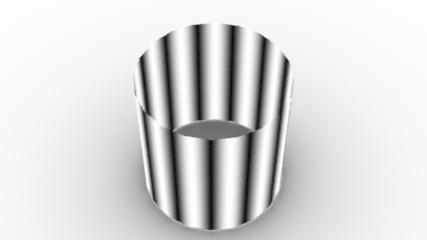Figure 1
Polygonal cylinder with well defined 'st' ('uv') coordinates.

Of course, if the shader is attached to geometry that has not been assigned texture coordinates the result of using 's' becomes problematic - figure 2.Figure 2
Polygonal cylinder with undefined texture coordinates.

Even more unpredictable are geometries that cannot be given texture coordinates. For example, the volumetric cylinder shown in figure 3 and the Blobby in figure 4.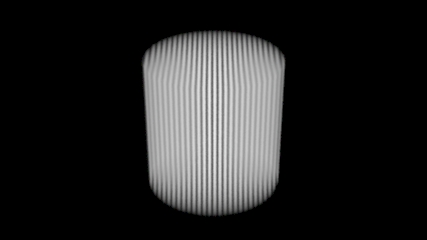Figure 3
Volumetric primitives cannot be assigned texture coordinates.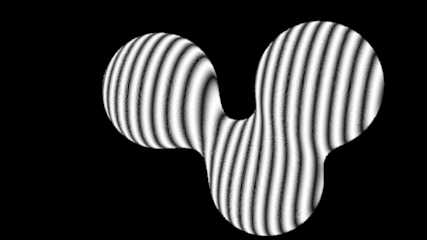Figure 4
Blobby primitives cannot be assigned texture coordinates

The next section demonstrates that shading according to 'xyz' position, rather than texture coordinates, generates a 3D solid texture.

#### A 3D Sinusoidal Texture

Rather than calculating a sine value based on a texture coordinate, such as 's', the polar coordinate of a shading point (generally a micro-polygon) can be used.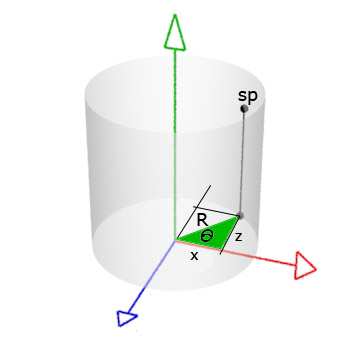Figure 5

For example, the angular position (theta) of `sp` shown in figure 5 can be specified by its 2D Polar coordinate. For the purpose of creating a pattern of vertical stripes the height of `sp` can be ignored. The shader given in listing 2 uses the sine pattern to control the final color (`Ci`) and final opacity (`Oi`).

Listing 2 (sine3d.sl)

 ```surface sine3d(float freq = 4, amp = 0.5, offset = 0, blur = 0.05) { // Convert the shading point to the object coordinate system. point p = transform("object", P); // Get the coordinates of the shading point. float x = p; float z = p; // The arc tangent of the shading point will be in radians // (-3.142 to 3.142) ie -180 to 180 degress. float theta = atan(x,z); // Generate a sine wave - remapped to 0 to 1. float wave = (sin(theta * freq) + 1) * 0.5; // Adjust the height and position of the sine wave. float waveHt = wave * amp + offset; // Get a filtered value of the wave height. waveHt = smoothstep(waveHt - blur, waveHt + blur, p); // Control the mixing of two colors by the waveHt. Oi = mix(color(1,1,1), color(0,0,0), waveHt); Ci = Oi * Cs * wave; }```

Figure 6 shows the sine3d shader assigned to a cylinder, a volume primitive and a blobby.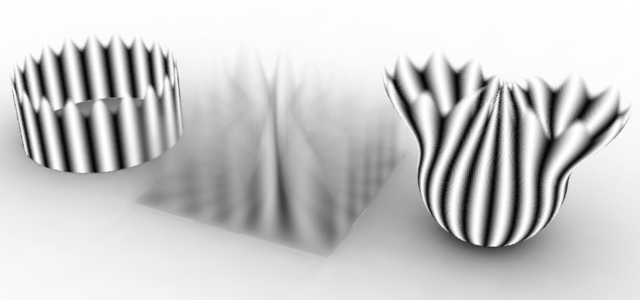Figure 6